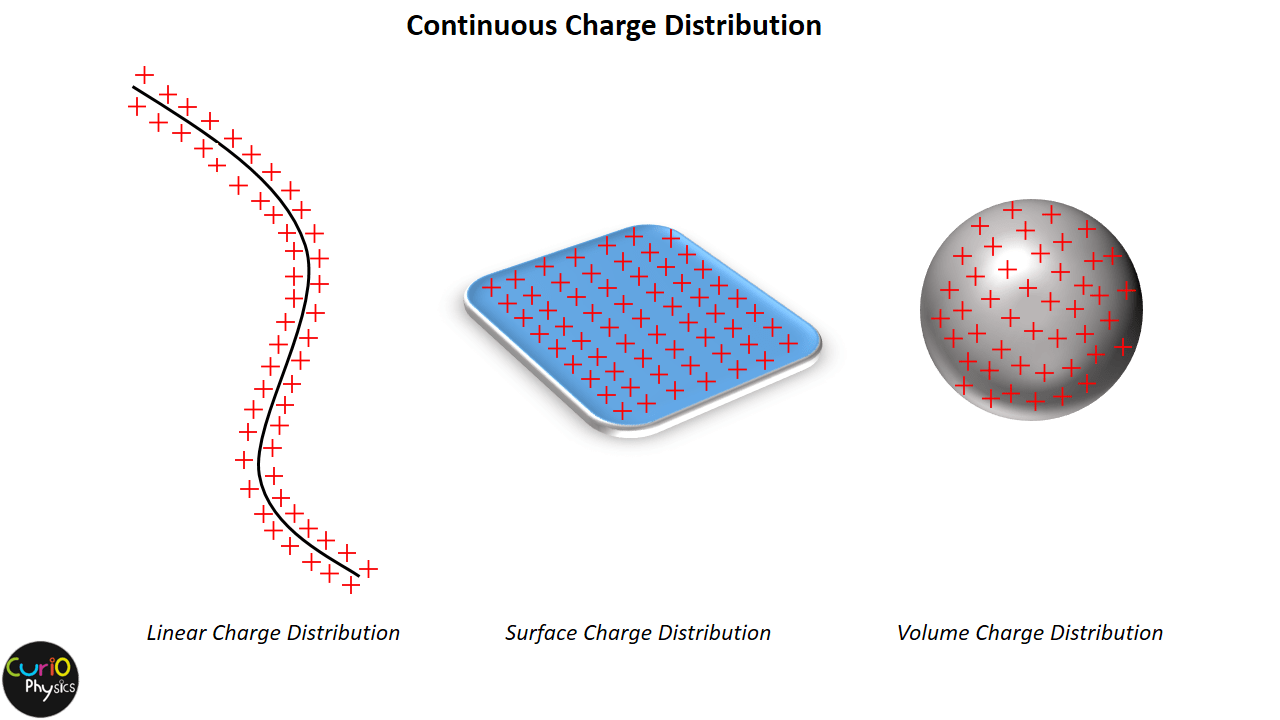# Electric Field Strength due to a Uniformly Charged Rod at a General Point

##### Electric Field Strength due to a Uniformly Charged Rod at a General Point

To find electric field strength at any general point P, let the point P be at a perpendicular distance R from the rod and let Q be the total charge (For specificity, Q is taken as positive, but the results apply to either sign of charge on the rod) on the rod. Let the linear charge density of the rod is λ (=Q/L).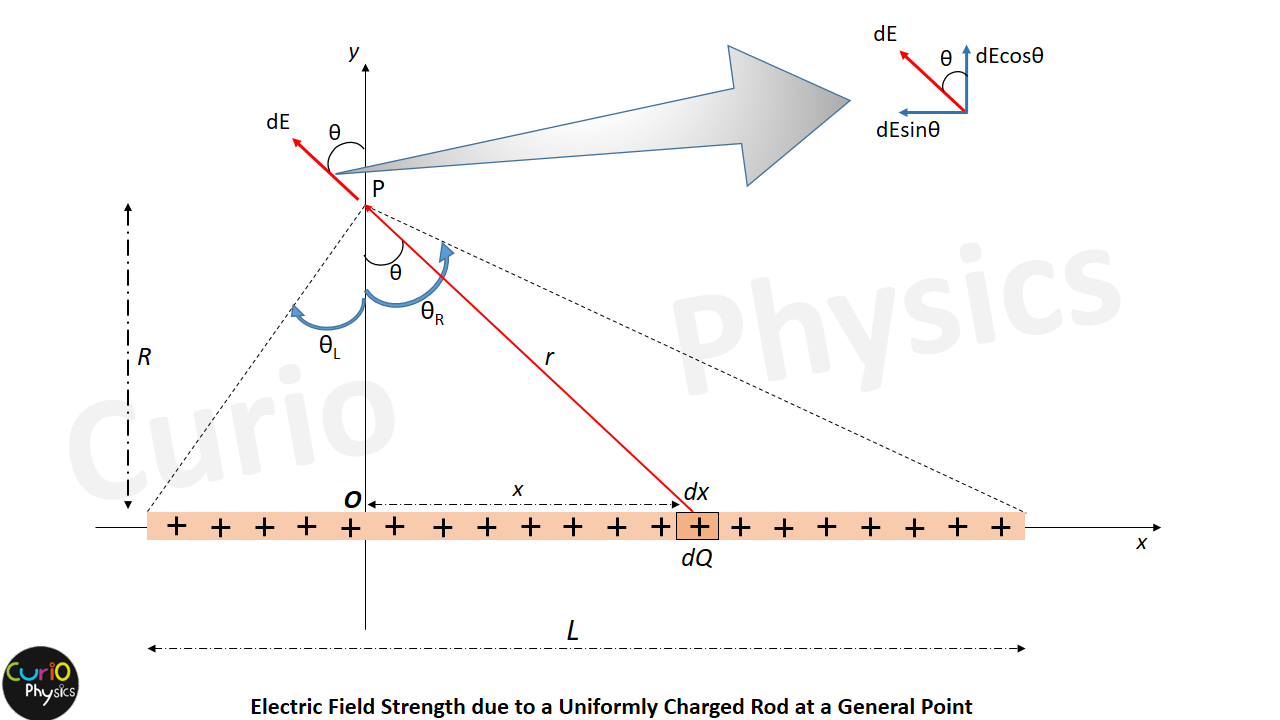The right end of the rod is located at angle θR measured counter-clockwise from the y axis and the left end of the rod is located at angle θL measured clockwise from the y axis. Using sign convention θR will be taken as positive and θL will be taken as negative.

To find electric field strength at P, we consider an element on rod of length dx at a distance x from point O as shown in figure. Now if dE is the electric field at P due to the element, then$\displaystyle dE=\frac{kdq}{{{r}^{2}}}$, here$\displaystyle dq=\frac{Q}{L}dx$ = λdx

The x component of the electric field is seen from this diagram will be :-$\displaystyle {{E}_{x}}=-\int{\frac{kdQ}{{{r}^{2}}}}\sin \theta =-\int{\frac{k\lambda dx}{{{r}^{2}}}}\sin \theta$     …..(1)

As dQ = λdx

[ Negative sign is because dEsinθ is in negative x-direction ]

and y component :-$\displaystyle {{E}_{y}}=\int{\frac{kdQ}{{{r}^{2}}}}\cos \theta =\int{\frac{k\lambda dx}{{{r}^{2}}}}\cos \theta$    …..(2)

Now from above figure x = R tanθ ⇒ dx = R sec2θ dθ    …..(3)

and r2 = R2 + x= R2 + (R tanθ)2 = R2 (1 + tan2θ) = R2sec2θ    …..(4)

Using the values of equations (3) and (4), in equations (1) and (2), we get$\displaystyle {{E}_{x}}=-\int{\frac{k\lambda (R{{\sec }^{2}}\theta d\theta )}{{{R}^{2}}{{\sec }^{2}}\theta }}\sin \theta =-\frac{k\lambda }{R}\int\limits_{{{\theta }_{L}}}^{{{\theta }_{R}}}{\sin \theta d\theta }$$\displaystyle {{E}_{x}}=-\frac{k\lambda }{R}(-\cos \theta )_{{{\theta }_{L}}}^{{{\theta }_{R}}}=\frac{k\lambda }{R}(\cos {{\theta }_{R}}-\cos {{\theta }_{L}})$    …..(5)

and similarly…$\displaystyle {{E}_{y}}=\int{\frac{k\lambda (R{{\sec }^{2}}\theta d\theta )}{{{R}^{2}}{{\sec }^{2}}\theta }}\cos \theta =\frac{k\lambda }{R}\int\limits_{{{\theta }_{L}}}^{{{\theta }_{R}}}{\cos \theta d\theta }$$\displaystyle {{E}_{y}}=\frac{k\lambda }{R}(sin\theta )_{{{\theta }_{L}}}^{{{\theta }_{R}}}=\frac{k\lambda }{R}(sin{{\theta }_{R}}-\sin {{\theta }_{L}})$    …..(6)

Note :- Equation (5) and (6) are the general results. The sign convention for θ is not applied till now. We will use these results in various interesting cases with sign convention for θ. Here are the different cases…..

Case I :- To find Electric Field Strength when the point P lies on the perpendicular bisector of the rod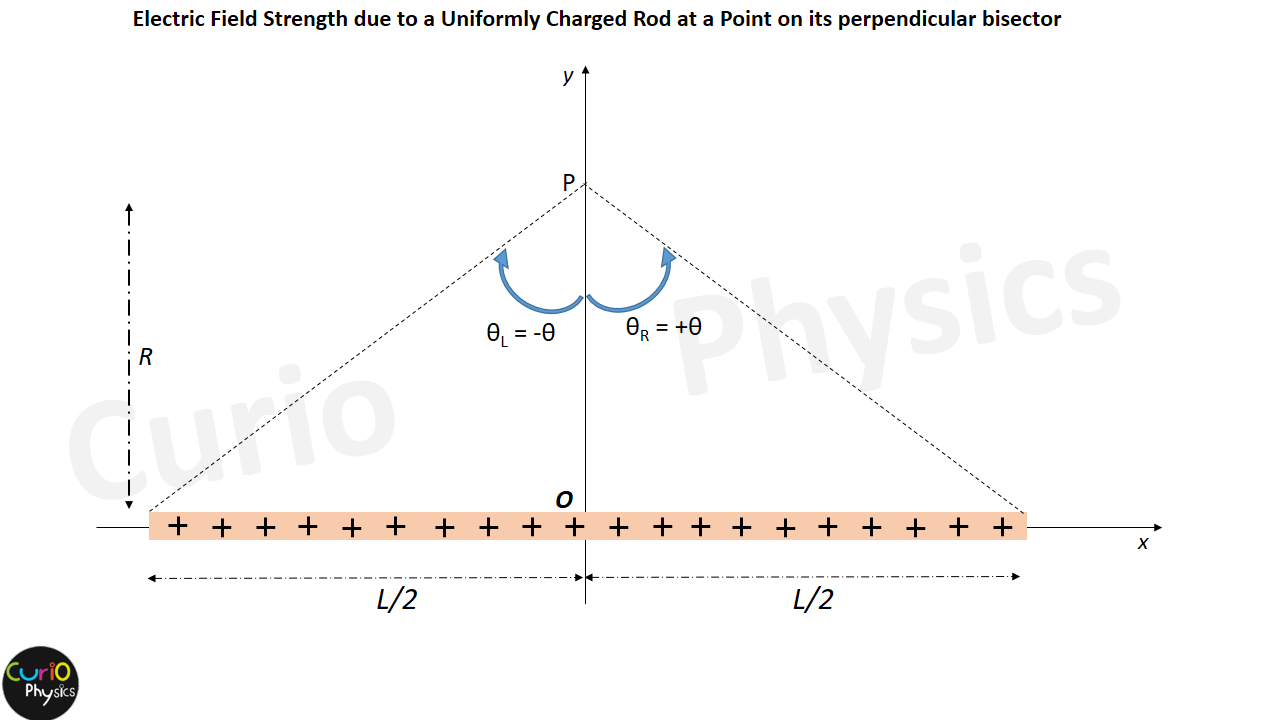On the perpendicular bisector of a the finite rod, θR = +θ and θL = -θ.

Then From Eq. (5), Ex = 0,  and Eq. (6) becomes$\displaystyle {{E}_{y}}=\frac{k\lambda }{R}[sin(+\theta )-\sin (-\theta )]=\frac{k\lambda }{R}(2\sin \theta )$$\displaystyle {{E}_{y}}=\frac{2k\lambda }{R}\frac{L/2}{\sqrt{{{R}^{2}}+{{(L/2)}^{2}}}}$

Or$\displaystyle {{E}_{y}}=\frac{kQ}{R\sqrt{{{R}^{2}}+{{L}^{2}}/4}}$    …..(7)

In this case also if R >>> L, then the result becomes$\displaystyle {{E}_{y}}=\frac{kQ}{{{R}^{2}}}$, this is nothing but Electric Filed Intensity due to a point charge.

Case II :- To find Electric Field Strength for an infinitely long rod

When the rod is of infinite length, then θR = +90° and θL = -90°.

From Eq. (5), Ex = 0,  and Eq. (6) gives$\displaystyle {{E}_{y}}=\frac{k\lambda }{R}[sin(+90)-\sin (-90)]=\frac{k\lambda }{R}(1+1)$$\displaystyle {{E}_{y}}=\frac{2k\lambda }{R}$    …..(8)

Case III :- To find Electric Field Strength when the point P lies exactly above the right end of the finite rodIn this case θR = +0° and θL = -θ°(Let).

Then equation (5) gives…$\displaystyle {{E}_{x}}=\frac{k\lambda }{R}[(\cos 0-\cos (-\theta )]=\frac{k\lambda }{R}(1-\cos \theta )$$\displaystyle {{E}_{x}}=\frac{k\lambda }{R}\left[ 1-\frac{R}{\sqrt{{{R}^{2}}+{{L}^{2}}}} \right]$    …..(9)

And equation (6) gives…$\displaystyle {{E}_{y}}=\frac{k\lambda }{R}[sin0-\sin (-\theta )]=\frac{k\lambda }{R}\sin \theta$$\displaystyle {{E}_{y}}=\frac{k\lambda }{R}\frac{L}{\sqrt{{{R}^{2}}+{{L}^{2}}}}$    …..(10)

Case IV :- To find Electric Field Strength when the point P lies exactly above the right end of a semi- infinite rod

In that case θR = +0° and θL = -90°.

Equation (5) gives…$\displaystyle {{E}_{x}}=\frac{k\lambda }{R}[(\cos 0-\cos (-90)]=\frac{k\lambda }{R}(1-0)$$\displaystyle {{E}_{x}}=\frac{k\lambda }{R}$    …..(11)

And from equation (6)…$\displaystyle {{E}_{y}}=\frac{k\lambda }{R}[sin0-\sin (-90)]=\frac{k\lambda }{R}(0+1)$$\displaystyle {{E}_{y}}=\frac{k\lambda }{R}$    …..(12)

Note that equations (11) and (12) gives Ex = Ey, which means that…

1. “electric field due to a charged rod of semi-infinite length at a point vertically above its right end (also true if point P lies vertically above the left end of semi-infinite rod), always points 45° away from the axis of the rod, regardless of how near or far the point is from the rod.” and
2. The value of equations (11) and (12) is half of the equation (8), which is obvious because if we join together the ends of two equally-charged semi-infinite rods, we get an infinite rod. The x components of the two rods are equal and opposite, so they cancel out and we get equation (8).

Case V :- To find Electric Field Strength when the point P lies vertically above a point S, which is at a distance d from the right end, on the axis of the finite charged rod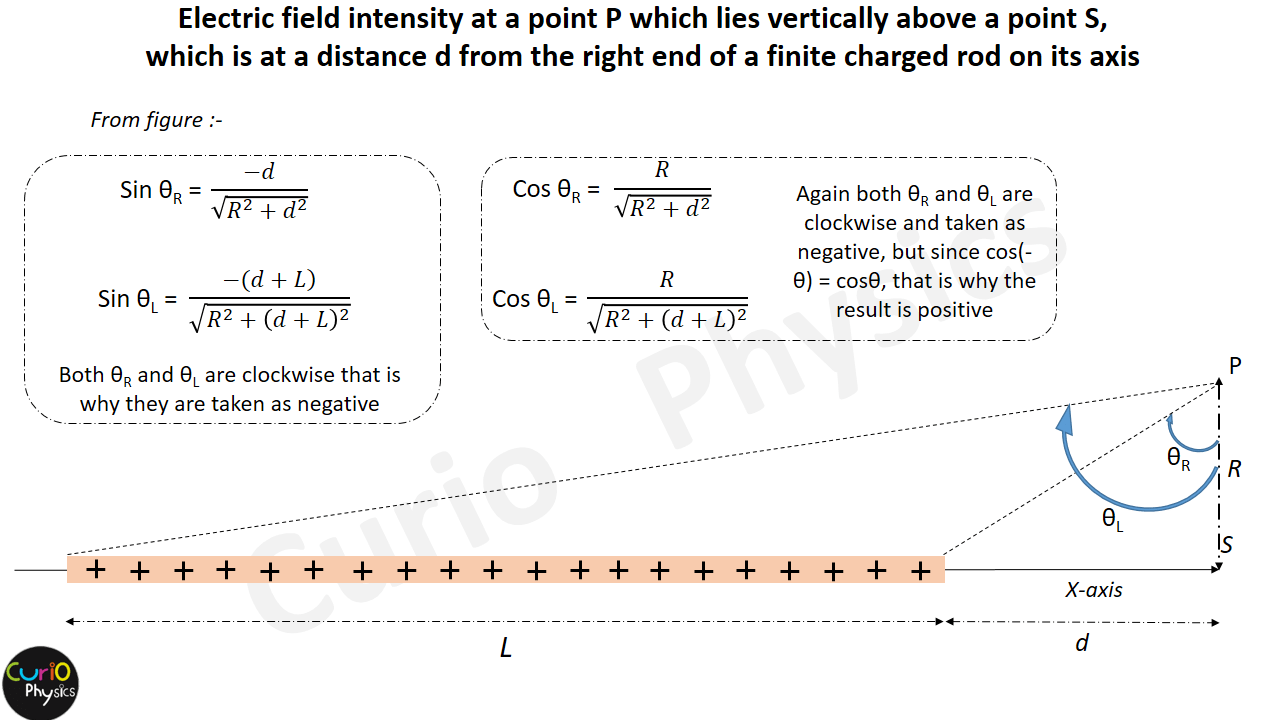From the above figure…$\displaystyle \sin {{\theta }_{R}}=\frac{-d}{\sqrt{{{R}^{2}}+{{d}^{2}}}}$$\displaystyle \sin {{\theta }_{L}}=\frac{-(d+L)}{\sqrt{{{R}^{2}}+{{(d+L)}^{2}}}}$$\displaystyle \cos {{\theta }_{R}}=\frac{R}{\sqrt{{{R}^{2}}+{{d}^{2}}}}$       and$\displaystyle \cos {{\theta }_{L}}=\frac{R}{\sqrt{{{R}^{2}}+{{(d+L)}^{2}}}}$

Using the value of cosθL and cosθR, in equation (5), we get…$\displaystyle {{E}_{x}}=\frac{k\lambda }{R}\left[ \frac{R}{\sqrt{{{R}^{2}}+{{d}^{2}}}}-\frac{R}{\sqrt{{{R}^{2}}+{{(d+L)}^{2}}}} \right]$$\displaystyle {{E}_{x}}=k\lambda \left[ \frac{1}{\sqrt{{{R}^{2}}+{{d}^{2}}}}-\frac{1}{\sqrt{{{R}^{2}}+{{(d+L)}^{2}}}} \right]$    …..(13)

Similarly, using the value of sinθL and sinθR, in equation (6), we get…$\displaystyle {{E}_{y}}=\frac{k\lambda }{R}\left[ \frac{-d}{\sqrt{{{R}^{2}}+{{d}^{2}}}}-\frac{-(d+L)}{\sqrt{{{R}^{2}}+{{(d+L)}^{2}}}} \right]$$\displaystyle {{E}_{y}}=\frac{k\lambda }{R}\left[ \frac{(d+L)}{\sqrt{{{R}^{2}}+{{(d+L)}^{2}}}}-\frac{d}{\sqrt{{{R}^{2}}+{{d}^{2}}}} \right]$    …..(14)

Case VI :- To find Electric Field Strength when the point P lies on the axis of the finite charged rod at a distance d from the right end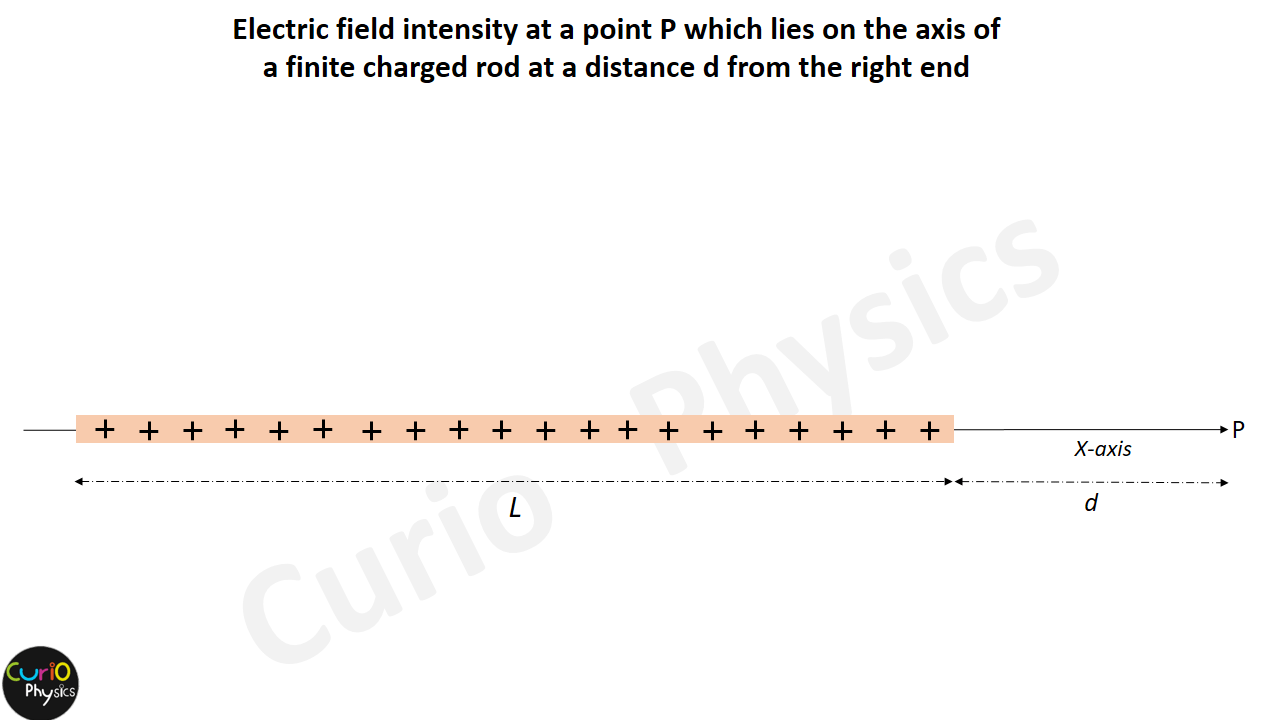From above figure as the point P lies on the axis of the rod so, R→0

Taking limit as R→0, sinθR and sinθR becomes….$\displaystyle \underset{R\to 0}{\mathop{\text{Lim}}}\,\left[ \sin {{\theta }_{R}}=\frac{-d}{\sqrt{{{R}^{2}}+{{d}^{2}}}} \right]\Rightarrow \sin {{\theta }_{R}}\to -1\text{ }$$\displaystyle \underset{R\to 0}{\mathop{\text{Lim}}}\,\left[ \sin {{\theta }_{L}}=\frac{-(d+L)}{\sqrt{{{R}^{2}}+{{(d+L)}^{2}}}} \right]\Rightarrow \sin {{\theta }_{L}}\to -1$

From equation (6), we get Ey = 0.

Similarly applying limit, R→0, in equation(5) we get…$\displaystyle \underset{R\to 0}{\mathop{Lim}}\,\left[ {{E}_{x}} \right]=\underset{R\to 0}{\mathop{Lim}}\,\left[ \frac{k\lambda }{R}\left[ \frac{R}{\sqrt{{{R}^{2}}+{{d}^{2}}}}-\frac{R}{\sqrt{{{R}^{2}}+{{(d+L)}^{2}}}} \right] \right]=\underset{R\to 0}{\mathop{Lim}}\,\left[ k\lambda \left[ \frac{1}{\sqrt{{{R}^{2}}+{{d}^{2}}}}-\frac{1}{\sqrt{{{R}^{2}}+{{(d+L)}^{2}}}} \right] \right]$$\displaystyle \Rightarrow {{E}_{x}}=k\lambda \left[ \frac{1}{d}-\frac{1}{d+L} \right]=\frac{k\lambda L}{d\left( d+L \right)}$$\displaystyle \Rightarrow {{E}_{x}}=\frac{kQ}{d\left( d+L \right)}$    …..(15)

[ Because λL = Q, total charge on the rod]

Note :- If d >>>>L, then, equation (15) becomes,$\displaystyle {{E}_{x}}=\frac{kQ}{{{d}^{2}}}$, which is nothing but Field Intensity due to a point charge Q at a distance d. Because if d >>>>L, then the rod will behave as a point charge.